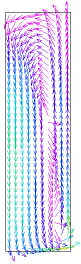﻿ Sample Problems > Applications > Fluids > swirl

# swirl

Navigation:  Sample Problems > Applications > Fluids >

# swirl{  SWIRL.PDE

 This problem addresses swirling flow in a cylindrical  vessel driven by a bottom impeller using a vector  description of the equations.  In two-dimensional cylindrical coordinates, we can  represent three velocity components (radial, axial  and tangential) as long as there is no variation of  cross-section or velocity in the azimuthal coordinate.  The Navier-Stokes equation for flow in an incompressible  fluid with no body forces can be written in FlexPDE  notation as    dens*(dt(U) + dot(U,grad(u)) = -grad(p) + visc*del2(U)    where U represents the vector fluid velocity, p is the  pressure, dens is the density and visc is the viscosity  of the fluid.  Here the pressure can be considered as  the deviation from static pressure, because uniform static  forces like gravity can be cancelled out of the equation.  In two-dimensional steady-state axisymmetric form, this  equation becomes three component equations, radial (vr),  tangential (vt) and axial (vz):    dens*(vr*dr(vr) - vt^2/r  + vz*dz(vr)) + dr(p) =                                visc*[div(grad(vr)) - vr/r^2]      dens*(vr*dr(vt) + vr*vt/r + vz*dz(vt)) =                                visc*[div(grad(vt)) - vt/r^2]      dens*(vr*dr(vz) + vz*dz(vz)) + dz(p) = visc*div(grad(vz))Notice that various strange terms arise, representing centrifugal and coriolis

forces in cylindrical coordinates and derivatives of the unit vectors in the

viscosity term. Notice also that there are no tangential derivatives, these

having been assumed zero.

In principle, these equations are supplemented by the equation of incompressible

mass conservation:

div(U) = 0

but this equation contains no reference to the pressure, which is nominally the

remaining variable to be determined.

In practice, we choose to solve a "slightly compressible" system by defining a

hypothetical equation of state

p(dens) = p0 + L*(dens-dens0)

where p0 and dens0 represent a reference density and pressure, and L is a large

number representing a strong response of pressure to changes of density.  L is

chosen large enough to enforce the near-incompressibility of the fluid, yet not

so large as to erase the other terms of the equation in the finite precision

of the computer arithmetic.

The compressible form of the continuity equation is

dt(dens) + div(dens*U) = 0

which, together with the equation of state yields

dt(p) = -L*dens0*div(U)

resulting in the final pressure equation:

In a real stirring vessel, the fluid is driven by an impeller bar in the bottom

of the fluid. Since we cannot directly represent this geometry in an axisymmetric

model, we approximate the effect of the impeller by a body force on the fluid

in the lower segment of the domain.  This body force attempts to accelerate the

fluid to the velocity of the stir bar, with an arbitrary partition of the

velocity into vr, vt and vz.

}

TITLE 'Swirling cylindrical flow'

COORDINATES

ycylinder ('r','z')

VARIABLES

V(0.001)  = vector(vr, vz, vt)

p(0.001) { pressure, with linear interpolation and minimum expected range  }

DEFINITIONS

ht=0.02     { vial height }

radwall = 0.95*rad { a gridding feature to put finer mesh at vial wall }

dens=1000     { fluid density }

visc=0.001   { fluid viscosity }

vm=magnitude(V)

div_v=  div(V)   { velocity divergence }

PENALTY = 1e4*visc/rad^2 { the phony equation of state coefficient }

band = ht/20 { height of force band }

bf = 1e5     { arbitrary body-force scaling }

f             { stirbar force - assigned by region later }

rpm =staged(10,20,30)   { several stirring speeds }

vimp = 2*pi*r*rpm/60     { impeller velocity }

vr0 = 0.2*vimp   { arbitrary partition of stirring velocity }

vt0 = 1.0*vimp

vz0 = 0.3*vimp

V0 = vector(vr0, vz0, vt0)

mass_balance = div_v/integral(1)

INITIAL VALUES

vr=0

vz=0

vt=0

p=0

EQUATIONS

BOUNDARIES

Region 'domain'

F=0

Start 'outer' (0,0)

{ mirror conditions on bottom boundary }

natural(vr)=0 natural(vt)=0   value(vz)=0     natural(p)=0   line to (rad,0)

{ no slip on sides (ie, velocity=0)  }

!      value(vt)=0     value(vz)=0

{ friction on sides  - generate an outward "Flux" of V (i.e. -visc*grad(V)) to drive it toward zero  }

natural(vt)=100*vt     natural(vz)=100*vz     natural(p)=0   line to (rad,ht)

{ zero pressure and no z-flow on top, but free vr and vt }

natural(vr)=0 natural(vt)=0   value(vz)=0     value(p)=0     line to (0,ht)

{ no radial or tangential velocity on spin axis }

value(vr)=0   value(vt)=0     natural(vz)=0   natural(p)=0   line to close

Region "impeller"

F=bf

{ add a gridding feature to help resolve the shear layer at the wall }

MONITORS

contour(vt) as "Swirling Velocity" report(rpm)

contour(vz) as "Axial Velocity" report(rpm)

elevation(vt,vt0) from(0,0) to (rad,0) as "Impeller Velocity" report(rpm)

contour(p) as "Pressure"

vector(vr,vz) as "R-Z Flow"

PLOTS

contour(vt) as "Swirling Velocity" report(rpm)

contour(vz) as "Axial Velocity" report(rpm)

contour(vm) as "Velocity Magnitude" report(rpm)

contour(p) as "Pressure" report(rpm)

vector(vr,vz) norm as "R-Z Flow" report(rpm)

contour(mass_balance) report(rpm)

elevation(vt) from (0,band) to (rad,band) as "Swirling Velocity" report(rpm)

elevation(vz) from (0,band) to (rad,band) as "Axial Velocity" report(rpm)

elevation(vt,vt0) from(0,band) to (rad,band) as "Impeller Velocity" report(rpm)

elevation(vm) from (0,band) to (rad,band) as "Velocity Magnitude" report(rpm)

elevation(vt) from (0,ht/2) to (rad,ht/2) as "Swirling Velocity" report(rpm)

elevation(vz) from (0,ht/2) to (rad,ht/2) as "Axial Velocity" report(rpm)

elevation(vm) from (0,ht/2) to (rad,ht/2) as "Velocity Magnitude" report(rpm)